2022 NL MVP Odds
+115
1.15 to 1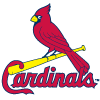Paul Goldschmidt
37.5% implied probability

+550
5.5 to 1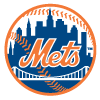Pete Alonso
12.4% implied probability

+550
5.5 to 1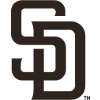Manny Machado
12.4% implied probability

+1200
12 to 1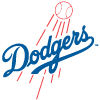Mookie Betts
6.2% implied probability

+1300
13 to 1Trea Turner
5.8% implied probability

+2500
25 to 1Freddie Freeman
3.1% implied probability

+3200
32 to 1Nolan Arenado
2.4% implied probability

+3500
35 to 1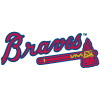Ronald Acuna
2.2% implied probability

+4000
40 to 1Francisco Lindor
2.0% implied probability

+5000
50 to 1Dansby Swanson
1.6% implied probability

+5000
50 to 1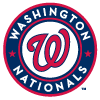Juan Soto
1.6% implied probability

+7000
70 to 1Austin Riley
1.1% implied probability

+8000
80 to 1Bryce Harper
1.0% implied probability

+8500
85 to 1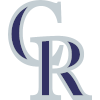C.J. Cron
0.9% implied probability

+11000
110 to 1Matt Olson
0.7% implied probability

+13000
130 to 1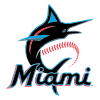Jazz Chisholm
0.6% implied probability

+15000
150 to 1Kyle Schwarber
0.5% implied probability

+18000
180 to 1Rhys Hoskins
0.4% implied probability

+18000
180 to 1Nick Castellanos
0.4% implied probability

+18000
180 to 1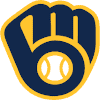Christian Yelich
0.4% implied probability

+18000
180 to 1Willy Adames
0.4% implied probability

+18000
180 to 1Corbin Burnes
0.4% implied probability

+18000
180 to 1Tommy Edman
0.4% implied probability

+25000
250 to 1Aaron Nola
0.3% implied probability

+25000
250 to 1Marcell Ozuna
0.3% implied probability

+25000
250 to 1Zack Wheeler
0.3% implied probability

+50000
500 to 1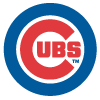Willson Contreras
0.2% implied probability

+50000
500 to 1Seiya Suzuki
0.2% implied probability

+50000
500 to 1Nelson Cruz
0.2% implied probability

+50000
500 to 1Jeff McNeil
0.2% implied probability

+50000
500 to 1Jake Cronenworth
0.2% implied probability

+50000
500 to 1Max Scherzer
0.2% implied probability

+50000
500 to 1Fernando Tatis
0.2% implied probability

+50000
500 to 1Dylan Carlson
0.2% implied probability

+50000
500 to 1Justin Turner
0.2% implied probability

+50000
500 to 1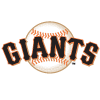Brandon Crawford
0.2% implied probability

+50000
500 to 1Bryan Reynolds
0.2% implied probability

+50000
500 to 1Charlie Blackmon
0.2% implied probability

+50000
500 to 1Jacob deGrom
0.2% implied probability

+50000
500 to 1Kris Bryant
0.2% implied probability

+50000
500 to 1Brandon Belt
0.2% implied probability

+50000
500 to 1Ke'Bryan Hayes
0.2% implied probability

+50000
500 to 1Trent Grisham
0.2% implied probability

+50000
500 to 1Walker Buehler
0.2% implied probability

+50000
500 to 1Cody Bellinger
0.2% implied probability

+50000
500 to 1Starling Marte
0.2% implied probability

+50000
500 to 1J.T. Realmuto
0.2% implied probability

+50000
500 to 1Josh Bell
0.2% implied probability

+50000
500 to 1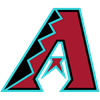Ketel Marte
0.2% implied probability

+50000
500 to 1Tyler O'Neill
0.2% implied probability

+50000
500 to 1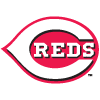Joey Votto
0.2% implied probability

+50000
500 to 1Ryan McMahon
0.2% implied probability